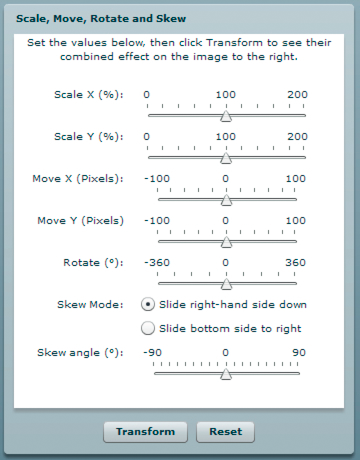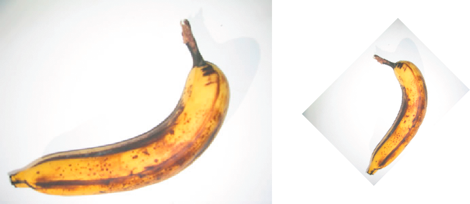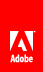# 几何形状示例：对显示对象应用矩阵转换

Flash Player 9 和更高版本，Adobe AIR 1.0 和更高版本

DisplayObjectTransformer 范例应用程序说明许多使用 Matrix 类来变换显示对象的功能，包括：

• 旋转显示对象

• 缩放显示对象

• 平移（重新定位）显示对象

• 倾斜显示对象DisplayObjectTransformer.mxml

DisplayObjectTransformer.fla

Flash (FLA) 或 Flex (MXML) 中的主应用程序文件

com/example/programmingas3/geometry/MatrixTransformer.as

img/

## 定义 MatrixTransformer 类

MatrixTransformer 类包含应用 Matrix 对象的几何变形的静态方法。

#### transform() 方法

transform() 方法包含以下属性的参数：

• sourceMatrix — 此方法转换的输入矩阵

• xScaleyScalexy 缩放系数

• dxdyxy 平移量（以像素为单位）

• rotation — 旋转量（以度为单位）

• skew — 倾斜系数（以百分比表示）

• skewType — 倾斜的方向，"right""left"

transform() 方法调用下列类的静态方法：

• skew()

• scale()

• translate()

• rotate()

#### skew() 方法

skew() 方法通过调整矩阵的 bc 属性来倾斜矩阵。可选参数 unit 确定用于定义倾斜角度的单位，如果必要，该方法会将 angle 值转换为弧度：

```if (unit == "degrees")
{
angle = Math.PI * 2 * angle / 360;
}
{
angle = Math.PI * 2 * angle / 100;
}```

`var skewMatrix:Matrix = new Matrix();`

skewSide 参数确定倾斜应用到的边。如果该参数设置为 "right"，则以下代码设置矩阵的 b 属性：

`skewMatrix.b = Math.tan(angle);`

`skewMatrix.c = Math.tan(angle);`

```sourceMatrix.concat(skewMatrix);
return sourceMatrix;```

#### scale() 方法

```if (percent)
{
xScale = xScale / 100;
yScale = yScale / 100;
}
sourceMatrix.scale(xScale, yScale);
return sourceMatrix;```

#### translate() 方法

translate() 方法只需通过调用矩阵对象的 translate() 方法即可应用 dxdy 平移系数，如下所示：

```sourceMatrix.translate(dx, dy);
return sourceMatrix;```

#### rotate() 方法

rotate() 方法将输入的旋转系数转换为弧度（如果提供的是角度或渐变），然后调用矩阵对象的 rotate() 方法：

```if (unit == "degrees")
{
angle = Math.PI * 2 * angle / 360;
}
{
angle = Math.PI * 2 * angle / 100;
}
sourceMatrix.rotate(angle);
return sourceMatrix;```

## 从应用程序中调用 MatrixTransformer.transform() 方法

```tempMatrix = MatrixTransformer.transform(tempMatrix,
xScaleSlider.value,
yScaleSlider.value,
dxSlider.value,
dySlider.value,
rotationSlider.value,
skewSlider.value,
skewSide );```

`img.content.transform.matrix = tempMatrix;`